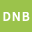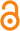Please use this identifier to cite or link to this item: `http://dx.doi.org/10.25673/33931`
 Title: Determining the cycle structure of permutation polynomials of shape x t + γTr (x k) Author(s): Gerike, Daniel Referee(s): Pott, AlexanderGranting Institution: Otto-von-Guericke-Universität Magdeburg, Fakultät für Mathematik Issue Date: 2020 Extent: V, 94 Seiten Type: HochschulschriftType: PhDThesis Exam Date: 2020 Language: English URN: urn:nbn:de:gbv:ma9:1-1981185920-341267 Subjects: KörperPolynome Abstract: In this thesis we study the cycle structure of permutation polynomials. They play an important role in many applications of finite fields. Of particular note are combinatorial design theory, cryptography and coding theory. An important application of permutation polynomials with known cycle structure in coding theory is their use as parts of turbo codes. In this context we want the permutations to be given as polynomials, because this reduces the implementation cost. But we also want to know their cycle structures, because they give us important algebraic and combinatorial properties of the permutations, which strongly in uence the performance of the final code. Currently we know the cycle structure only for a few simple classes of permutation polynomials. These are monomials, Dickson and linearized polynomials. We give a survey of these results. None of these classes make full use of the structure of a finite field and mainly depend on either the multiplicative or additive group of the field. We focus on permutation polynomials of shape Xt+ Trqn=q(Xk) 2 Fqn[X], where 2 Fqn and 1 t; k qn-1. In contrast to those classes, for which the cycle structure is already known, these depend on both the additive and multiplicative structure of a finite field, but still have a nice algebraic form. Permutation polynomials of this shape were first considered in 2008 by Charpin and Kyureghyan  for q = 2, where a complete classification was given. We show, that polynomials of shape Xt+ f(X), where f : Fqn -> Fq, can only be permutations if gcd(t; qn - 1) = 1. In this case Xt is also a permutation, so for classification purposes it suffces to consider t = 1. In recent years Kyureghyan and Zieve , Ma and Ge  and Li, Qu, Chen, and Li  have constructed 24 infinite families of permutation polynomials of shape X + Trqn=q(Xk). We give the number of fixed points for all of these permutations. Further we show that permutation polynomials of shape X + f(X), where f : Fqn -> Fq, are precisely those that also permute any line + Fq, 2 Fqn. We give a condition on f, under which certain of these induced permutations on lines have the same cycle structure. All 17 of the 24 infinite families, where n is fixed to 2 or 3 satisfy this condition. In particular, if n = 2, which holds for 15 of them, this allows us to ascertain the cycle structure by determining it on Fq and any one other line + Fq. The cycle structure on Fq can be found easily, but getting the cycle structure on one of the other lines is still a very difficult problem. We solve it for two families completely and for one family in a special case. URI: https://opendata.uni-halle.de//handle/1981185920/34126http://dx.doi.org/10.25673/33931 Open Access:Open access publication License:(CC BY-SA 4.0) Creative Commons Attribution ShareAlike 4.0 Appears in Collections: Fakultät für Mathematik